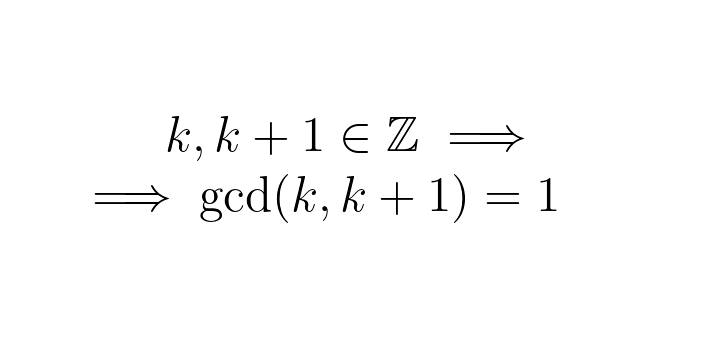The Python 🐍 problem-solving bootcamp 🚀 is starting soon. Join the second cohort now!

## Twitter proof: consecutive integers are coprime

Let's prove that if $$k$$ is an integer, then $$\gcd(k, k+1) = 1$$. That is, any two consecutive integers are coprime.